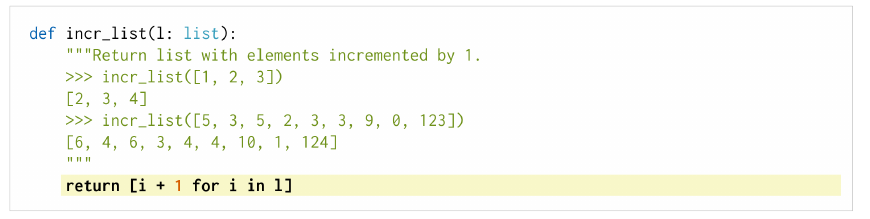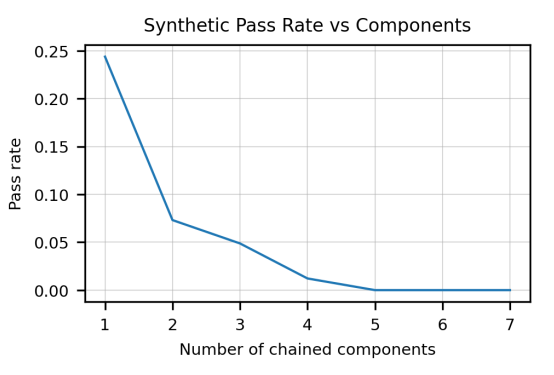• Natural Language Processing
• 🞄
• Introductions
• 🞄
• Theory & Algorithms

# OpenAI Codex: Why the revolution is still missing

Fabian GringelIn this blog post, I'll explain how Codex from OpenAI works, and in particular how it differs from GPT-3. I will outline why I think it should be used with caution and is not ready yet to revolutionize the software development process.

## Introduction

If you have spent some time writing code, you might have experienced that even complex software can (and should) be broken down into smaller units (e.g. functions) performing generic tasks. Writing these smaller pieces can be quite a nuisance: even though it is often neither especially challenging nor interesting, writing them is time consuming and keeps you from focusing on the more delicate parts of the software development process.

This is where OpenAI's Codex comes to the rescue: Codex promises to write simple functions based on natural language descriptions of their functionality. As a developer, you would only have to write the function signature and doc string and Codex would take care of its implementation.

What Codex does is not really new: it's just a language model specialized on writing code. In fact, it is a direct descendant of GPT-3. If you have never heard of GPT-3 or you are not sure what it is all about, then I recommend you skim over this introductory blog post before you go on.

## Codex vs. GPT-3

Codex is a fine-tuned version of the fully trained GPT-3. Hence we should have a look at

1. which data was used for fine-tuning Codex,

2. how the performance between the two differs.

### Fine-tuning Datasets

In order to fine-tune Codex, OpenAI collected a dataset of public GitHub repositories, which totaled 159 GB. This code base was then used as a "text" corpus to train Codex on the language modeling task "predict the next word".

In addition, they created a dataset of carefully selected training problems which mirror the evaluation task (see below) more closely than the code found on GitHub, which they used for further fine tuning.

### Codex' Performance

To evaluate how well Codex can write Python functions on its own, OpenAI created a data set of 164 programming problems called HumanEval. Each problem consists of a function (always including a doc string) and a comprehensive set of unit tests.

Codex is presented with a prompt containing only the function signature and the doc string, and it's job is then to complete the function such that it passes all unit tests (passing all unit tests counts as a success, failing at least one, as a failure).Prompt with white, (correct) completion with yellow background. Image taken from Chen et al 2021.

In order to measure performance, a pass@k metric is used, where k is an integer: For every problem in the HumanEval data set, we let Codex produce k different outputs (e.g. k=1, k=10 or k=100). The problem counts as solved if at least one of the outputs passes all unit tests. The pass@k value is then the fraction of problems that were solved.

In case you wonder how we can get k different completions for one and the same prompt and not k times the same completion: The completions are generated probabilistically, in particular at each step (i.e. the generation of the next word) we do not just choose the most probable next word, but sample from Codex' probability distribution for the next word (that is, the most probable next word has only the highest chance of being chosen as the next word). This introduces randomness.

However, the pass@k evaluation set-up might appear not very suitable to assess the usefulness of Codex in a real world situation: Assume we calculate the pass@k metric for a k>>1, say k=100. Then, even if the resulting score is high, the only thing you know about the performance is that Codex returns the correct answer once in a while, possibly after many unsuccessful tries. But can you rely on it in practice?

First of all, you should probably not rely blindly on code produced by some black box algorithm like Codex anyway, even if the reported metrics were outstanding. This applies to the code you write yourself too, hence it is often suggested to use unit tests. But unit tests become even more useful when you want Codex to assist you: Just let Codex generate a multitude of completions, and then filter out all of them which do not pass the unit tests. If you have written enough unit tests, after filtering you are left with only those functions that perform the desired task. So for practical purposes it might suffice that Codex is correct "once in a while".

Here is Codex' performance in comparison to the best-performing of all tested GPT-models.

 pass@1 pass@100 Codex-S 12B 37.7% 77.5% GPT-J 6B 11.62% 27.74%

## Working with Codex

Currently there are two ways to use Codex: either through OpenAI's API (coming with a convenient user interface called "Playground"), which I was lucky to be able to try out, or through GitHub Copilot, an IDE extension that continuously suggests completions. Currently, there are waiting lists for both of them.

If you want to get a feeling for what Codex is able to do, then I recommend watching the OpenAI Codex live demo:

I agree that Codex is astonishing. However, here I rather want to focus on the limitations of Codex and its actual practical value.

### Limitations

In the paper introducing Codex, OpenAI points out two important limitations:

1. There is a negative correlation between complexity of the doc string and the pass rate. The doc string complexity is quantified as the number of "chained components" it contains, where a single component is an instruction like “convert the string to lowercase”. In other words: Codex is only good at writing simple functions (see image below).

2. Codex is not trained to generate high quality code, but to recreate the "average" code found at GitHub. This means that Codex adopts and perpetuates widespread bad coding habits. (This issue is analogous to language models perpetuating stereotypes present in their training data.)The performance of Codex-12B deteriorates drastically with more complex doc strings.

These two limitations have implications for how Codex should be used in practice: First, it is necessary to break down the desired functionality into simple functions that Codex can handle.

Doing this is still quite an effort and often not trivial - certainly human software developers won't become obsolete in the near future.

Second, even if Codex manages to generate functions that pass all unit tests - if you are interested in not only in having some code that works, but concise, efficient and secure code, then you should double check what Codex is outputting. In some cases this might take more time than actually writing the code yourself (or copying it from StackOverflow).

### Anchoring and Automation Bias

In a blog post dedicated to Codex, Jeremy Howard points out another type of problem: anchoring and automation bias. As humans, we have a tendency to rely overly on the first piece of information we encounter and on automated suggestions - even if we are aware of the dangers and try to be skeptical. Both biases apply to Codex-suggested code, meaning: even if we know Codex' suggestions are off very often, we will still have a tendency to rely on them.

### An Example Case

Consider the following function:

def count_right_numeric(cell_indices: Tuple[int, int],
df: pd.DataFrame) -> int:
"""Counts how many cells containing numbers are to the right of a given \
cell.

Args:
cell_indices (Tuple[int, int]): Indices of a cell.
df (pd.DataFrame): DataFrame containing the cell.

Returns:
int: Number of numeric cells.
"""
row, col = cell_indices
numeric_count = 0
for i in range(col + 1, len(df.columns)):
if df.iloc[row, i] is not None:
if isinstance(df.iloc[row, i], str) \
and df.iloc[row, i].isnumeric():
numeric_count += 1
elif isinstance(df.iloc[row, i], float) \
or isinstance(df.iloc[row, i], int):
numeric_count += 1
return numeric_count

The signature and the doc string are taken from a function that we used in a real project (slightly reworded, though). Given a Pandas DataFrame and a cell within it, specified by its indices, we want to know the number of cells in the same row, but on the right of the given cell which contain only numbers.

The body of the function above was indeed generated by Codex ("best of 20" with temperature 0.5, first try) - and does just what it should. (Admittedly, there is some ambiguity in the doc string instruction - what does it mean exactly to "contain numbers"? Codex decides for "contain only numbers" - which was intended.)

Now we can be happy with what Codex gives us and go on. Or we can wonder whether Codex' implementation is really the best solution to the problem. The following two lines of code also perform the task, without the cumbersome for-loop and case distinctions:

    right_of_cell = df.iloc[cell_indices, cell_indices + 1:]
return pd.to_numeric(right_of_cell, errors="coerce").notnull().sum()

Due to the effects of anchor and automation bias, we might be inclined to stick to Codex' suggestions, even if there are better alternatives at hand. This would result in worse code. (Which of the two function bodies above is to be preferred depends of course on the context and also on taste.)

In general, it seems to me that Codex' completions are often too lengthy and don't make use of handy built-in functions like to_numeric.

## Conclusion

Codex is a fascinating tool that showcases the current state of deep learning. For practical purposes, however, it should be used with caution, since using Codex might actually make you write worse code.